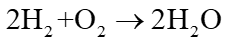Choose if the given statement is true/false.The composition of water is two atoms of hydrogen with one atom of oxygen (H2O). They combine in the ratio of 2:1.

# Choose if the given statement is true/false.The composition of water is two atoms of hydrogen with one atom of oxygen (H2O). They combine in the ratio of 2:1.

1. A
True
2. B
False

Fill Out the Form for Expert Academic Guidance!l

+91

Live ClassesBooksTest SeriesSelf Learning

Verify OTP Code (required)

### Solution:

The given statement is true.
Concept:  We already know that no matter how many water molecules are extracted, the chemical composition of water will always have the same proportion of hydrogen to oxygen atoms. Water is having formula.
Every time hydrogen and oxygen gas are combined to form H2O, two molecules of diatomic hydrogen gas and one molecule of oxygen gas are required in order to create two molecules of water. The following chemical reaction takes place:In other words, based on the above chemical reaction, we can infer that the proportions of hydrogen to oxygen are 2:1, hydrogen to water are 1:1, and oxygen to water are 1:2.
Hence, the correct answer is option 1.+91

Live ClassesBooksTest SeriesSelf Learning

Verify OTP Code (required)